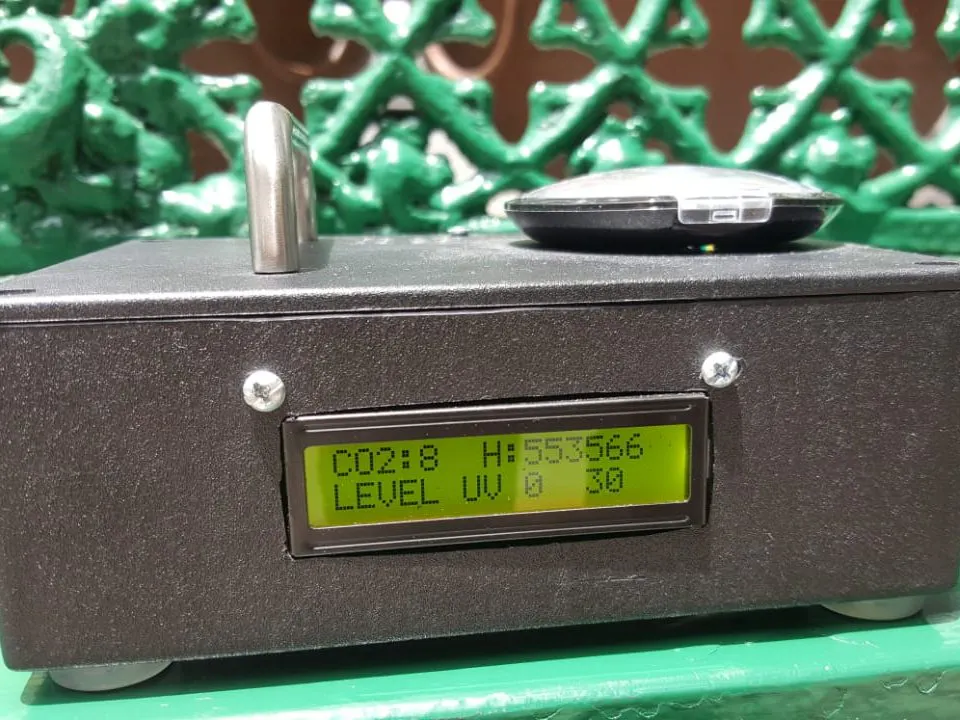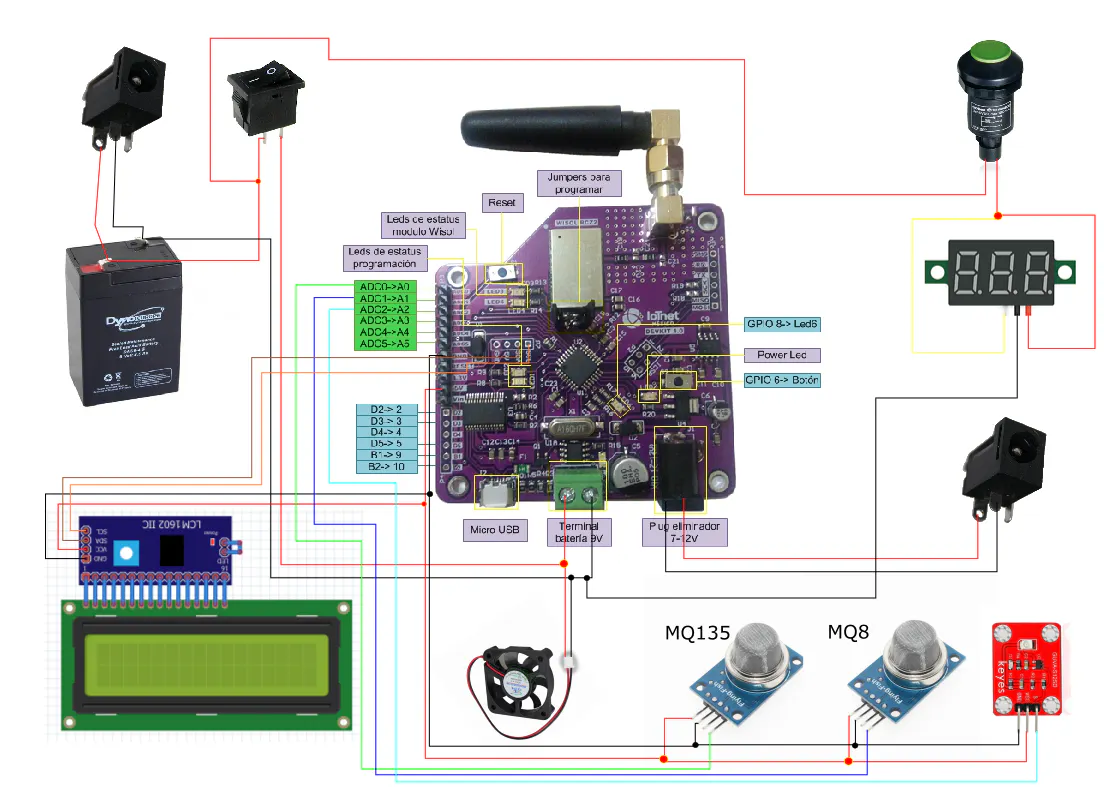Published

# Arduino Sigfox: School Warden to Monitor the Environment

This project is for monitor the air and UV conditions of the environment before the children go to recess.

IntermediateWork in progressOver 2 days1,104## Things used in this project

### Hardware components

 DevKit Sigfox NXTIoT
×1
 6 Volt 4.5 Ah Sealed Lead Acid Rechargeable Battery -
×1
×1
 Adafruit i2c / SPI character LCD backpack
×1Altoids tin A SMALL BOX TO PROTEC THE UV SENSOR
×1
 MQ135 Gas Sensor for Air Qaulity (ER-SEN17850M) Digital / Analog output
×1
 MQ8 Gas Sensor for Hydrogen
×1
 UV sensor
×1
 DC Power Monitor DC 0-100 V Voltage Meter LED green
×1
 Medium Orange Plastic Mechanical Push Button
×1
 Switch button 2 positions
×1
 4 in. (102mm) Satin Nickel Wire Drawer Pull
×1

### Software apps and online servicesArduino IDESigfoxUbidots

### Hand tools and fabrication machines

 Variety Screwdriver Set (6-Piece)
 4g Ultra Gel Control Super Glue Bottle or a hot glu gun
 Hammer
 5.5-Amp Corded 3/8 in. Variable Speed Compact Drill/Driver with Bag
 Dremel
 8 in. Wire Stripper and Cutter and Crimper
 Electric welding pencil

## Custom parts and enclosures

### All the dimentions from the proyect

you just need to unzip the file an the open with catia or with solidworks

## Schematics

### Schematic## Code

### Code for school warden

Arduino
```/* PROJECT: THE SCHOOL WARDEN WITH SIGFOX
* AUTOR: LUIS ARENAS
* EMAIL: WICHOMW3@HOTMAIL.COM
*
*/
#include <LiquidCrystal_I2C.h> // library for the I2C DISPLAY
#include<Wire.h> // library for the comunication by I2C

#define PIN_GUVAS12SD A2 // Pin al que se conecta el módulo con el UVM30A
#define ESPERA_ENTRE_LECTURAS 100 // Leer cada 100 ms // Read every 100 ms
#define ESPERA_ENTRE_PRESENTACIONES 10000 // Mostrar el índice cada 10 s // show every 10 seconds the average
#define CANTIDAD_INDICES_UV 12 // cuantity of UV levels *you can change it
#define CANTIDAD_INDICES_CO2 6 // cuantity of CO2 levels *you can change it

LiquidCrystal_I2C lcd(0x3F,16,2); // define the I2C Direction (Direction in hexadecimal,witdh,height)

unsigned int lectura_sensor; //variable to read the analog value of uv sensor
unsigned int contador_lecturas=1; // counter of lectures for uv sensor
unsigned int contador_lecturas2=1; // counter of lectures for MQ135
float total_lecturas=0.0; // the sum of all lectures from uv sensor
float media_lecturas;   // keep the average from the uv sensor values
float total_lecturas_mq135=0.0; // the sum of all the lectures from MQ135
float media_lecturas_mq135; // the average form the MQ135 values
int valor_indice_uv[CANTIDAD_INDICES_UV]={0,210,295,378,467,563,646,738,818,907,1003,1022}; // ranges of Uv values from 0 to 11 * you can change it
int valor_indice_CO2[CANTIDAD_INDICES_CO2]={0,8,11,16,19,23};  // ranges of CO2 from 0 to 6 * you can change it
byte indice; // variable to keep the number of the array of UV
byte indice2; // variable to keep the number of the array of CO2
boolean buscando_indice_uv; // Varible that turns true when the average of uv is greater than some value of the uv array
boolean buscando_indice_CO2; // Varible that turns true when the average of CO2 is greater than some value of the CO2 array
long cronometro_lecturas; // take the time that has been passed between lectures
long cronometro_presentaciones; // take the time that has been passed when the info is showed
long tiempo_transcurrido; // take the time that have been passed

const int boton=6;//default pin for the boton just for this model
const int led=7; // default pin for the LED just for this model
char RespuestaSigfox; // The String for the message
const int mq135=A0; // define the analog pin for the MQ135 sensor
const int mq8=A1; // define the analog pin for the MQ8 sensor

int sensor1=0; // variable to keep the value of MQ135 and add to the message for sigfox
int sensor2=0; //variable to keep the value of MQ8 and add to the message for sigfox
int sensor3=0; //variable to keep the value of UV and add to the message for sigfox

float sensor11=0; // variable to read the analog value of MQ135
float sensor22=0; // variable to read the analog value of MQ8

int ban=0;
long RO=68760; // value obtained by the operation with a rule of three with the video
float RS_mq135=0; // value of the resistence S for MQ135
float RS_mq8=0; // value of the resistence S for MQ8
int ppm_mq135=0; // value of ppm for MQ135 * you can change it to long if you want
long ppm_mq8=0; // value of ppm for MQ8
float var11; // value of divide RS from MQ8 with RO
float var22; // value of divide RS from MQ135 with RO

void setup() {

Serial.begin(9600); // begin the port Serial in the arduino
pinMode(boton, INPUT); // define the pin boton like input
pinMode(7, OUTPUT);  // define the pin LED like output
pinMode(mq135,INPUT); // define the analog pin for MQ135
pinMode(mq8,INPUT);  // define the analog pin for MQ8
//pinMode(PIN_GUVAS12SD,INPUT); // La lectura analógica no necesita inicialización
lectura_sensor=analogRead(PIN_GUVAS12SD); // The first lecture is an error
cronometro_lecturas=millis(); // wait one round of read to stabilize the sensor
cronometro_presentaciones=millis();

// define a welcome message
lcd.init();
lcd.backlight();
lcd.clear();
lcd.setCursor(0,0);
lcd.print("WELCOME");
lcd.print(" SIGFOX");
delay(3000);
lcd.clear();
lcd.setCursor(0,0);
lcd.print("Processing info");
lcd.setCursor(0,1);
}

void enviar_sigfox(int x, int y, int z) // this funtion converts he 3 values of the sensors to hexadecimal and add to the sigfox message to send it
{
String bufer="AT\$SF=";
Serial.print("Enviar/send: ");
Serial.println(x);
Serial.println(y);
Serial.println(z);
//convierte el dato a bytes y lo agrega a nuestro mensaje a enviar
//str1=  String(sensor1, HEX);

//
digitalWrite(7, HIGH);
delay(1000);
enviarcomandoATSigfox("AT");
enviarcomandoATSigfox("AT\$RC");
digitalWrite(7, LOW);
}

void loop() {

tiempo_transcurrido=millis()-cronometro_lecturas;

if(tiempo_transcurrido>ESPERA_ENTRE_LECTURAS) // read every time that the millis pass the value stablish in the top
{
cronometro_lecturas=millis();
lectura_sensor=(lectura_sensor*1100)/225.06; // converts the value to de real value in volts from the sensor, because the sensor just reach lectures of 1.1 volts

//to calculate the value of MQ135
sensor11=(sensor11*5.0)/1023.0;
RS_mq135=1000*((5-sensor11)/sensor11);
var22=RS_mq135/RO;
ppm_mq135=5.0908*(pow(var22,-0.343));

total_lecturas_mq135+=ppm_mq135; //to sum the value of MQ135 to a variable
total_lecturas+=lectura_sensor; // to sum the valur of UV to a variable

}
tiempo_transcurrido=millis()-cronometro_presentaciones;
if(tiempo_transcurrido>ESPERA_ENTRE_PRESENTACIONES) // if the millist are greater than the time to prresente the total
{ // substract all the information of the ranges
cronometro_presentaciones=millis();
buscando_indice_uv=true;
buscando_indice_CO2=true;
while(buscando_indice_uv&&indice>0) // look for the value from the greatest to the lowest
{
indice--;
if(media_lecturas>valor_indice_uv[indice]) // if the average is higher than that value readed
{

buscando_indice_uv=false;  //active the flag
}
}
while(buscando_indice_CO2&&indice2>0) // if the average is higher than that value readed
{
indice2--;
if(media_lecturas_mq135>valor_indice_CO2[indice2])
{

buscando_indice_CO2=false; // active the flag
}
}

// if you want to see the values in the serial  monitor but if you are using sigfox you must be to desactivated
/*Serial.print("Lectura sensor: "+String(media_lecturas,DEC));
Serial.print (" Lectura de mq135: "+String(media_lecturas_mq135,DEC)+"");
Serial.print (" sensor de mq135: "+String(analogRead(mq135),DEC)+"");
Serial.print (" sensor de mq8: "+String(analogRead(mq8),DEC)+"");
Serial.println(" Índice UV: "+String(indice,DEC));
Serial.println(" Índice CO2: "+String(indice2,DEC));
*/
//-----------------------------------------------------

lcd.clear(); // clean the display
sensor22=(sensor22*5.0)/1023.0; // transform the analog value into voltage by a rule of three
RS_mq8=1000*((5-sensor22)/sensor22); // this operation is for obtain RS value - the value of 1000 is a constant obtained by the resisitence between  voltage and analog port
var11=RS_mq8/RO; // a pre division of the final operation
ppm_mq8=20163*(pow(var11,-1.438)); // this operation is the equation obtained by excel

// show the results on the display
lcd.setCursor(0,0);
lcd.print("CO2:");
lcd.print((int)media_lecturas_mq135);

lcd.setCursor(7,0);
lcd.print("H:");
lcd.print(ppm_mq8);

lcd.setCursor(12,1);
lcd.print((int)media_lecturas);
// this switch is for know the value of the place in the array of UV sensors
switch (indice){
case 1:
lcd.setCursor(0,1);
lcd.print("LEVEL UV 1");
sensor3=18;
break;
case 2:
lcd.setCursor(0,1);
lcd.print("LEVEL UV 2");
sensor3=19;
break;
case 3:
lcd.setCursor(0,1);
lcd.print("LEVEL UV 3");
sensor3=20;
break;
case 4:
lcd.setCursor(0,1);
lcd.print("LEVEL UV 4");
sensor3=21;
break;
case 5:
lcd.setCursor(0,1);
lcd.print("LEVEL UV 5");
sensor3=22;
break;
case 6:
lcd.setCursor(0,1);
lcd.print("LEVEL UV 6");
sensor3=23;
break;
case 7:
lcd.setCursor(0,1);
lcd.print("LEVEL UV 7");
sensor3=24;
break;
case 8:
lcd.setCursor(0,1);
lcd.print("LEVEL UV 8");
sensor3=25;
break;
case 9:
lcd.setCursor(0,1);
lcd.print("LEVEL UV 9");
sensor3=26;
break;
case 10:
lcd.setCursor(0,1);
lcd.print("LEVEL UV 10!!");
sensor3=27;
break;
case 11:
lcd.setCursor(0,1);
lcd.print("LEVEL UV 11!!!");
sensor3=28;
break;
case 0:
lcd.setCursor(0,1);
lcd.print("LEVEL UV 0");
sensor3=17;
break;
}
// this switch is for know the place of the array of the MQ135 sensor
switch (indice2){
case 1:
sensor1=18;
break;
case 2:
sensor1=19;
break;
case 3:
sensor1=20;
break;
case 4:
sensor1=21;
break;
case 5:
sensor1=22;
break;
case 0:
sensor1=17;
break;
}
// this part is for have a range for the MQ8 sensor
if(ppm_mq8<300000){
sensor2=17;
}

else if(ppm_mq8>300000 && ppm_mq8<360000){
sensor2=17;
}
else if(ppm_mq8>450000 && ppm_mq8<850000){
sensor2=18;
}
else if(ppm_mq8>950000 && ppm_mq8<1500000){
sensor2=19;
}
else if(ppm_mq8>1500000){
sensor2=19;
}

//restar the counters and the average for MQ135 and UV sensor
total_lecturas=0.0;
total_lecturas_mq135=0.0;
}

if (digitalRead(boton)==LOW) // if the boton is pressed we send the values to sigfox
{
enviar_sigfox(sensor1,sensor2,sensor3);
delay(2000);
}
}

void enviarcomandoATSigfox(char* comandoAT){ //tis funtion call the sigfox module to send the message
unsigned long x=0;
x = 0;
memset(RespuestaSigfox, '\0',sizeof(RespuestaSigfox));
Serial.print(comandoAT);
Serial.print("\r\n");
while(true){
if(Serial.available() != 0){
x++;
if (strstr(RespuestaSigfox, "\n") != NULL){
break;
}
}
}
}
```

## Credits

### Luis Eduardo Arenas Deseano

2 projects • 3 followers
I am a mechatronic, in communications and electronics engineer. Specialist in IoT, mobile conectivity and service robotics.

### Japhet Lopez

1 project • 2 followers